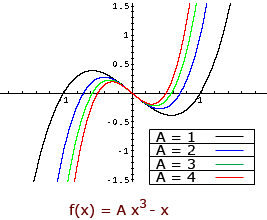Subject: Parameters Name: Chase Who are you: Student (All) What is the meaning of the word "parameters" when used in reference to Algebra. Hi Chase, The mathematical meaning of parameter according to the Oxford English Dictionary is A quantity which is fixed (as distinct from the ordinary variables) in a particular case considered, but which may vary in different cases; esp. a constant occurring in the equation of a curve or surface, by the variation of which the equation is made to represent a family of such curves or surfaces. Thus for example in the expression f(x) = A x3 - x x is the variable and A is a parameter. The function f(x) represents a family of curves that depend on the parameter A.Penny